# Class 6 Maths NCERT Solutions for Chapter 11 Algebra Ex – 11.5

## Algebra

Question 1.
State which of the following are equations (with a variable). Give reason for your answer. Identify the variable from the equations with a variable.

(a) 17 = x + 7
(b) (t – 7) > 5
(c)$\frac { 4 }{ 2 }$ = 2
(d) 7 x 3 – 19 = 8
(e) 5 x 4 – 8 = 2x
(f) x – 2 = 0
(g) 2m < 30
(h) 2n + 1 = 11
(i) 7 = (11 x 5) – (12 x 4)
(j) 7 = 11 x 2 + p
(k) 20 = 5y
(l)$\frac { 3q }{ 2 }$ < 5
(m) z + 12 > 24
(n) 20 – (10 – 5) = 3 x 5
(o) 7 – x = 5

Solution:
(a) An equation, equation with variable (x).
(b) Not an equation (as have no = sign).
(c) An equation, equation with numbers.
(d) An equation, equation with numbers.
(e) An equation, equation with variable (x).
(f) An equation, equation with variable (x).
(g) Not an equation (as have no = sign).
(h) An equation, equation with variable (n).
(i) An equation, equation with numbers.
(j) An equation, equation with variable (p).
(k) An equation, equation with variable (y).
(l) Not an equation (as have no = sign).
(m) Not an equation (as have no = sign),
(n) An equation, equation with numbers.
(o) An equation, equation with variable (x).

Question 2.
Complete the entries in the third column of the table.

Solution:
The table duly completed is as under :Question 3.
Pick out the solution from the values given in the bracket next to each equation. Show that the other values do not satisfy the equation.

(a) 5m = 60 (10, 5, 12, 15)
(b) n + 12 = 20 (12, 8, 20, 0)
(c) p – 5 = 5 (7, 2, 10, 14)
(d)$\frac { q }{ 2 }$ = 7 (4, -2, 8, 0)
(e) r – 4 = 0 (4, -4, 8, 0)
(f) x + 4 = 2 (-2, 0, 2, 4)

Solution:
(a) Given equation is 5m = 60.
For m=10: 5m = 5 x 10 = 50 ≠ 60. So, m=10 does not satisfy the given equation.
For m = 5 5m = 5 x 5 = 25 ≠ 60. So, m = 5 does not satisfy the given equation.
For m=12: 5m = 5 x 12 = 60. So, m=12 does not satisfy the given equation.
Thus, n = 12 is its solution.
For m = 15 5m = 5 x 15 = 75 ≠ 60. So, m = 15 does not satisfy the given equation.

(b) Given equation is n +12 = 20.
For n=12: n +12 = 12 + 12 = 24 ≠ 20. So, n = 12 does not satisfy the the given equation.
For n = 8: n +12 = 8 + 12 = 20. So, n = 8 satisfies the given equation.
Thus, n = 8 is its solution.
For n = 20: n + 12 = 20 + 12 = 32 ≠ 20. So, n = 20 does not satisfy the given equation.
For n = 0: n + 12 = 0 + 12 = 12 ≠ 20. So, n = 0 does not satisfy the given equation.

(c) Given equation is p – 5 = 5.
For p = 0: p – 5 = 0 – 5 = -5 ≠ 5. So, p = 0 does not satisfy the given equation.
For p = 10: p – 5 = 10 – 5 = 5. So, p = 10 satisfies the given equation.
Thus, its solution is p = 10.
For p = 5: p – 5 = 5 – 5 = 0≠ 5. So, p = 5 does not satisfy the given equation.
For p = -5: p – 5 = -5 – 5 = -10 ≠ 5. So, p = -5 does not satisfy the given equation.

(d) Given equation is$\frac { q }{ 2 }$ = 7.
For q = 7:$\frac { q }{ 2 }$ =$\frac { 7 }{ 2 }$ ≠ 7. So, q = 7 does not satisfy the given equation.
For q = 2:$\frac { q }{ 2 }$ =$\frac { 2 }{ 2 }$ = 1 ≠ 7. So, q = 2 does not satisfy the given equation.
For q = 10:$\frac { q }{ 2 }$ =$\frac { 10 }{ 2 }$ = 5 ≠ 7. So, q = 10 does not satisfy the given equation.
For q = 14:$\frac { q }{ 2 }$ =$\frac { 14 }{ 2 }$ = 7. So, q = 14 satisfies the given equation. Thus, q = 14 is its solution.

(e) Given equation is r – 4 = 0.
For r = 4: r – 4 = 4 – 4 = 0. So, r = 4 satisfies the given equation. Thus, r = 4 is its solution.
For r = -4: r – 4 = -4 – 4 = 8 ≠ 0. So, r = -4 does not satisfy the given equation.
For r = 8: r – 4 = 8 – 4 = 4 ≠ 0. So, r-8 does not satisfy the given equation.
For r = 0: r – 4 = 0 – 4 = -4 ≠ 0. So, r = 0 does hot satisfy thh given equation.

(f) Given equation is x + 4 = 2.
For x = -2: x + 4 = -2 + 4 = 2. So, x = -2 satisfies the given equation.
Thus, x = -2 is its solution.
For x = 0: x + 4 = 0 + 4 = 4 ≠ 2. So,- x =0 does not satisfy the given equation.
For x=2: x + 4= 2 + 4= 6 ≠ 2. So, x = 2 does not satisfy the given equation.
For x = 4: x + 4 = 4 + 4= 8 ≠ 2. So, x = 4 does not satisfy the given equation.

Question 4.
Question (a)
Complete the table and by inspection of the table find the solution to the equation m +10 = 16.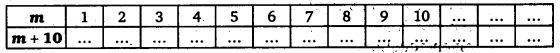Solution:
Completing the table, we have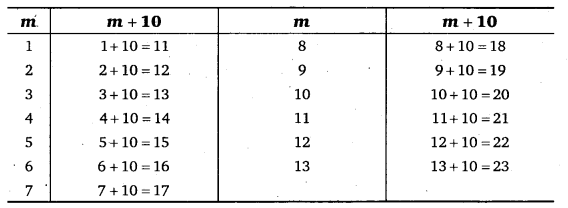By inspection of the above table, we find that m = 6 satisfies the equation m+10 = 16. So, m = 6 is its solution.

Question (b)
Complete the table and by inspection of the table find the solution to the equation 51 = 35.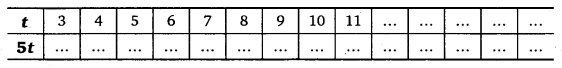Solution: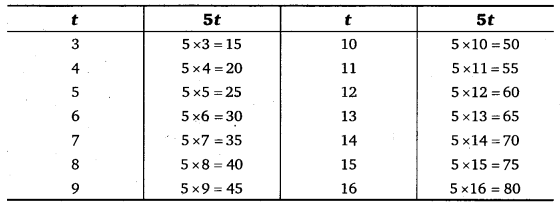By inspection of the above table, we find that t = 7 satisfies the equation 5t = 35. So, t = 7 is its solution.

Question (c)
Complete the table and find the solution of the equation$\frac { z }{ 3 }$ = 4 using the table.Solution:
Completing the table, we haveBy inspection of the above table, we find that t=12 satisfies the equation$\frac { z }{ 3 }$ = 4. So, t = 12 is its solution.

Question (d)
Complete the table and find the solution to .the equation m – 7 = 3.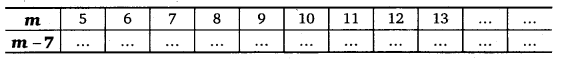Solution:
Completing the table, we have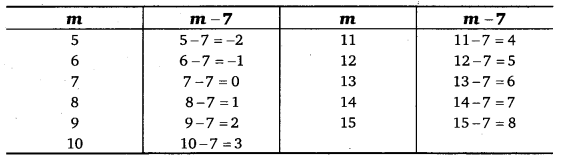By inspection of the above table, we find that m = 10 satisfies the equation m – 7 = 3. So, m = 10 is its solution.

Question 5.
Solve the following riddles, you may yourself construct such riddles.

Who am I?

(i)
Go round a square
Counting every comer Thrice and no more!
To get exactly thirty four!

(ii) For each day of the week
Make an upcount from me
If you make no mistake
You will get twenty three!

(iii) I am a special number
Take away from me a six!
A whole cricket team
You will still be able to fix!

(iv) Tell me who I am
I shall give a pretty clue!
You will get me hack
If you take me out of twenty two!

Solution:
(i) Let I be denoted by x. There are 4 comers of a square.
On counting each comer thrice, we get 3 x 4 = 12
As per problem:
x + 12 = 34 ⇒ x +12 – 12 = 34 – 12
⇒ x + 0 = 22 ⇒ x = 22
Thus, I am 22.

(ii) According to the question:
x + 7 =23
x + 7 – 7 = 23 – 7
⇒ x + 0 =16 ⇒ x =16

(iii) Let the special number be x. Then, according to the problem, we have
x – 6 = 11 [ In a cricket team, no. of players =11]
⇒ x – 6 + 6 = 11 + 6
⇒ x + 0 = 17 ⇒ x = 17
Thus, the special number is 17.

(iv) Let I be denoted by x.
According to the question:
22 – x = x
⇒ 22 – x + x = x + x
⇒ 22 + 0 =2x
⇒ 2x = 22 ⇒$\frac { 2x }{ 2 } =\frac { 22 }{ 2 }$
⇒ x =11
Thus, I am 11.